##### Pre-Calculus For Dummies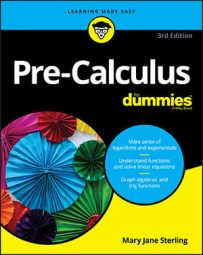Almost every function has an inverse. An inverse function basically undoes a function. The trigonometric functions sine, cosine, and tangent all have inverses, and they're often called arcsin, arccos, and arctan.

In trig functions, theta is the input, and the output is the ratio of the sides of a triangle. If you're given the ratio of the sides and need to find an angle, you must use the inverse trig function: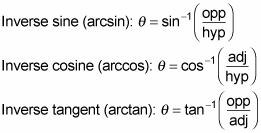Here's what an inverse trig function looks like in action. To find the angle theta in degrees in a right triangle if the tanθ = 1.7, follow these steps:

1. Isolate the trig function on one side and move everything else to the other.

This step is done already. Tangent is on the left and the decimal 1.7 is on the right: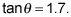2. Isolate the variable.

You're given the ratio for the trig function and have to find the angle. To work backward and figure out the angle, use some algebra. You have to undo the tangent function, which means using the inverse tangent function on both sides: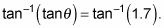This equation simplifies to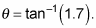3. Solve the simplified equation.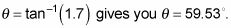Read the problem carefully so you know whether the angle you're looking for should be expressed in degrees or radians. Set your calculator to the correct mode.